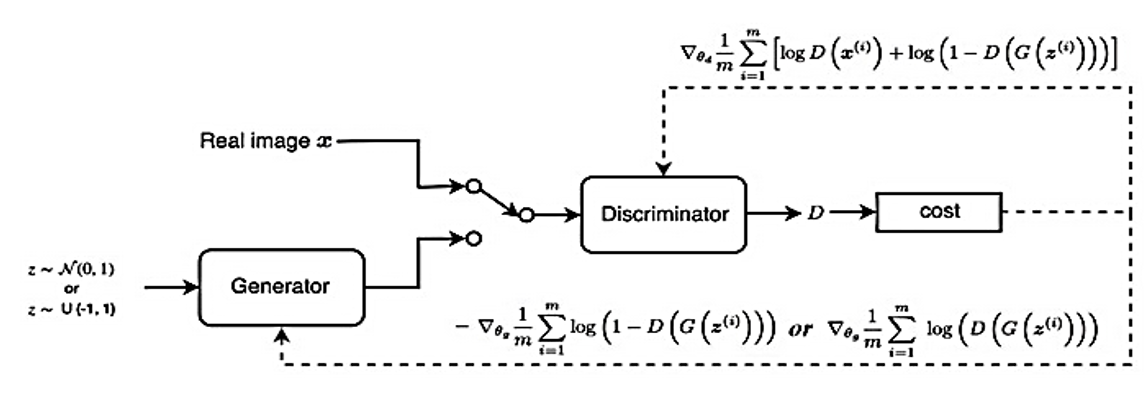# The Problems of Generative Adversarial Networks (GANs)In the last post I really discussed GANs (the structure and the steps of the training and the loss function). In this post, I will discuss the limitation and the problem of training a GAN.

In the last post I really discussed GANs (the structure and the steps of the training and the loss function). In this post, I will discuss the limitation and the problem of training a GAN.

## 1. Introduction

There are many various problems that prevent successful GAN training due to the GANs’ extremely diverse applications. Accordingly, improving the training of the GANs is an open research field for researchers.

Before discussing the problems, let have a quick look at some of the GAN equations.The architecture of the GAN with derivation terms of the loss function

The figure above indicates, the derivation terms of the final loss function for training the discriminator and generator with the corresponding gradient. In the following are some of the basic problems of GAN that lead to hard training.

The first problem in the GAN training that should be considered serious is vanishing gradients. Before diving into the Vanishing gradients problem, the KL-divergence and JS-divergence should be explained.

The concept of divergence between two probability distributions can be defined as a measure of the distance between two distributions. Imagine if we minimize the divergence, we also hope that the two distributions are equal.

### 2.1 KL-Divergence

In many generative models, the goal is to create a model which maximizes the Maximum Likelihood Estimation(shortly MLE) which can be defined as the best model parameters to fit the training data. Maximum likelihood equation is shown in follow.

## The Vanishing/Exploding Gradient Problem in Deep Neural Networks

Understanding the obstacles that faces us when building deep neural networks. A difficulty that we are faced with when training deep Neural Networks is that of vanishing or exploding gradients.

## Solving the Vanishing Gradient Problem with Self-Normalizing Neural Networks using Keras

In this post, we will learn how to create a self-normalizing deep feed-forward neural network using Keras. This will solve the gradient instability issue, speeding up training convergence, and improving model performance.

## Introduction to Neural Networks

There has been hype about artificial intelligence, machine learning, and neural networks for quite a while now. This will not be a math-heavy introduction because I just want to build the idea here.

## Neural network: what is a neural network?

Neural networks, as their name implies, are computer algorithms modeled after networks of neurons in the human brain. Learn more about neural networks from Algorithmia.

## A Comparative Analysis of Recurrent Neural Networks

Recurrent neural networks, also known as RNNs, are a class of neural networks that allow previous outputs to be used as inputs while having hidden states.